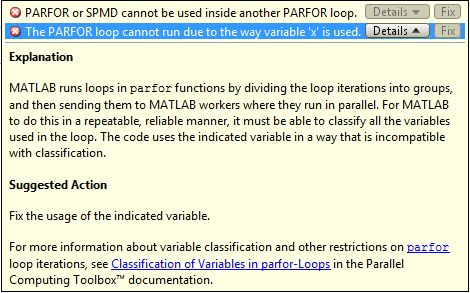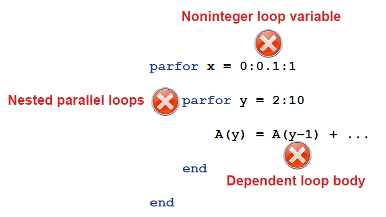Documentation

## Convert `for`-Loops Into `parfor`-Loops

In some cases, you must modify the code to convert `for`-loops to `parfor`-loops. This example shows how to diagnose and fix `parfor`-loop problems using a simple nested `for`-loop. Run this code in MATLAB® and examine the results.

```for x = 0:0.1:1 for k = 2:10 x(k) = x(k-1) + k; end x end```

To speed up the code, try to convert the `for`-loops to `parfor`-loops. Observe that this code produces errors.

```parfor x = 0:0.1:1 parfor k = 2:10 x(k) = x(k-1) + k; end x end```

In this case you cannot simply convert the `for`-loops to `parfor`-loops without modification. To make this work, you must change the code in several places. To diagnose the problems, look for Code Analyzer messages in the MATLAB Editor.This code shows common problems when you try to convert `for`-loops to `parfor`-loops.To solve these problems, you must modify the code to use `parfor`. The body of the `parfor`-loop is executed in a parallel pool using multiple MATLAB workers in a nondeterministic order. Therefore, you have to meet these requirements for the body of the `parfor`-loop:

1. The body of the `parfor`-loop must be independent. One loop iteration cannot depend on a previous iteration, because the iterations are executed in parallel in a nondeterministic order. In the example,

```x(k) = x(k-1) + k; ```
is not independent, and therefore you cannot use `parfor`. For next steps in dealing with independence issues, see Ensure That parfor-Loop Iterations are Independent.

2. You cannot nest a `parfor`-loop inside another `parfor`-loop. The example has two nested `for`-loops, and therefore you can replace only one `for`-loop with a `parfor`-loop. Instead, you can call a function that uses a `parfor`-loop inside the body of the other `parfor`-loop. However, such nested `parfor`-loops give you no computational benefit, because all workers are used to parallelize the outermost loop. For help dealing with nested loops, see Nested parfor and for-Loops and Other parfor Requirements.

3. `parfor`-loop variables must be consecutive increasing integers. In the example,

```parfor x = 0:0.1:1 ```
has non-integer loop variables, and therefore you cannot use `parfor` here. You can solve this problem by changing the value of the loop variable to integer values required by the algorithm. For next steps in troubleshooting `parfor`-loop variables, see Ensure That parfor-Loop Variables Are Consecutive Increasing Integers.

4. You cannot break out of a `parfor`-loop early, as you can in a `for`-loop. Do not include a return or break statement in the body of your `parfor`-loop. Without communication, the other MATLAB instances running the loop do not know when to stop. As an alternative, consider `parfeval`.

If you still have problems converting `for`-loops to `parfor`-loops, see Troubleshoot Variables in parfor-Loops.

### Tip

You can profile a `parfor`-loops using `tic` and `toc` to measure the speedup compared to the corresponding `for`-loop. Use `ticBytes` and `tocBytes` to measure how much data is transferred to and from the workers in the parallel pool. For more information and examples, see Profiling parfor-loops.